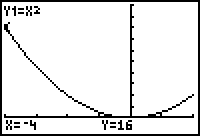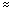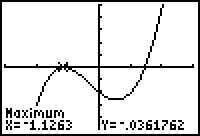# Extreme Values of Functions

Lesson 1

13.1.1

As shown in the previous example, the function has only one critical point, which occurs at x = 0. The values of the output at the critical point and at the endpoints are f(-4) = 16, f(0) = 0 and f(2) = 4.

The function has:

1. an absolute and a local maximum of 16 at x = -4,
2. an absolute and a local minimum of 0 at x = 0, and
3. a local maximum of 4 at x = 2.

The graph below, shown in a [-4, 2, 1] x [-2, 20, 2] window, supports the conclusions.Lesson 2
13.2.1 The local maximum is approximately y = -0.036 when x-1.126. The local minimum is approximately y = -2.639 when x0.559.

Lesson 3
13.3.1

The first derivative of the function is f'(x) = 3x2 - 3. The critical points are x = -1 and x = 1 because f'(-1) = 0 and f'(1) = 0.

The second derivative of the function is f"(x) = 6x. The second derivative evaluated at the critical points yields f"(-1) < 0 and f"(1) > 0.

Therefore, f has a local maximum at x = -1 and a local minimum at x = 1. The y-value at the local maximum is 7, and the y-value at the local minimum is 3. These are found by evaluating f(-1) and f(1) respectively.

Lesson 4
13.4.1The local maximum occurs at approximately (-1.1263, -0.0362). Depending on what you select for the bounds and the initial guess, the x-coordinate shown on your screen might differ slightly from the one shown in the graph above.

There is a critical point at x = 0 because the first derivative is undefined there. The endpoints are at x = -1 and x = 2.

The absolute maximum of 4 occurs at x = 0.

The absolute and local minimum of approximately 2.680 occurs at x = 2.

A local minimum of 3 occurs at x = -1.

A local minimum of approximately -0.688 occurs at x0.955.